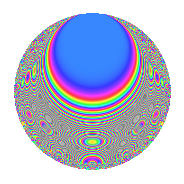# Properties

 Label 315.2.ceLevel 315 Weight 2 Character orbit ce Rep. character $$\chi_{315}(53,\cdot)$$ Character field $$\Q(\zeta_{12})$$ Dimension 64 Newform subspaces 1 Sturm bound 96 Trace bound 0

# Related objects

## Defining parameters

 Level: $$N$$ = $$315 = 3^{2} \cdot 5 \cdot 7$$ Weight: $$k$$ = $$2$$ Character orbit: $$[\chi]$$ = 315.ce (of order $$12$$ and degree $$4$$) Character conductor: $$\operatorname{cond}(\chi)$$ = $$105$$ Character field: $$\Q(\zeta_{12})$$ Newform subspaces: $$1$$ Sturm bound: $$96$$ Trace bound: $$0$$

## Dimensions

The following table gives the dimensions of various subspaces of $$M_{2}(315, [\chi])$$.

Total New Old
Modular forms 224 64 160
Cusp forms 160 64 96
Eisenstein series 64 0 64

## Trace form

 $$64q + 8q^{7} + O(q^{10})$$ $$64q + 8q^{7} + 8q^{10} + 32q^{16} - 48q^{22} - 16q^{25} + 88q^{28} + 32q^{31} - 16q^{37} - 40q^{40} - 16q^{43} - 80q^{52} - 32q^{55} - 88q^{58} + 48q^{61} - 32q^{67} - 112q^{70} - 88q^{73} - 320q^{76} - 56q^{82} + 16q^{85} + 120q^{88} - 128q^{91} + 208q^{97} + O(q^{100})$$

## Decomposition of $$S_{2}^{\mathrm{new}}(315, [\chi])$$ into newform subspaces

Label Dim. $$A$$ Field CM Traces $q$-expansion
$$a_2$$ $$a_3$$ $$a_5$$ $$a_7$$
315.2.ce.a $$64$$ $$2.515$$ None $$0$$ $$0$$ $$0$$ $$8$$

## Decomposition of $$S_{2}^{\mathrm{old}}(315, [\chi])$$ into lower level spaces

$$S_{2}^{\mathrm{old}}(315, [\chi]) \cong$$ $$S_{2}^{\mathrm{new}}(105, [\chi])$$$$^{\oplus 2}$$

## Hecke Characteristic Polynomials

There are no characteristic polynomials of Hecke operators in the database